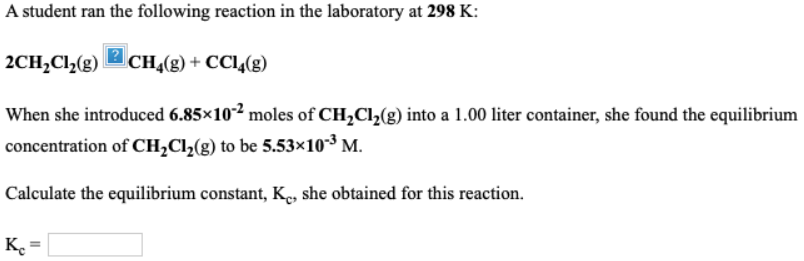# A student ran the following reaction in the laboratory at 298 K: 2CH2Cl2(g) -> CH4(g) + CCl4(g) When she introduced 6.85 x 10^-2 moles of CH2Cl2(g) into a 1.00 liter container, she found the equilibrium concentration of CH2Cl2(g) to be 5.53 x 10^-3 M. Calculate the equilibrium constant, Kc, she obtained for this reaction. Kc = ___